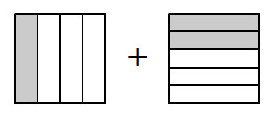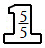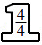### Home > MC2 > Chapter 1 > Lesson 1.2.5 > Problem1-110

1-110.

Mario is trying to explain to Marissa how to add fractions. He has written the work below.

1. Why did Mario start by rewriting the fractions using a Giant One? Why did he choose $20$ as his new denominator?

$\frac{1}{4}+\frac{2}{5}$Refer to the Math Notes box in Lesson 1.2.4. The denominators need to be the same to add the fractions.

2. Finish the problem. Explain each step you would take, and why.

Step 1:

$\frac{1}{4}·$$=\frac{5}{20}$          $\frac{2}{5}·$$=\frac{8}{20}$

Now that the denominators are the same, try solving the problem by adding the numerators.